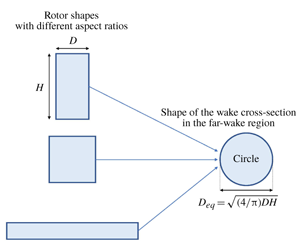Hostname: page-component-7d8f8d645b-r82c8 Total loading time: 0 Render date: 2023-05-28T13:23:22.077Z Has data issue: false Feature Flags: { "useRatesEcommerce": true } hasContentIssue false

## AbstractVariability of the rotor aspect ratio is one of the inherent characteristics of vertical-axis wind turbines (VAWTs) which differentiates them especially from the more conventional horizontal-axis wind turbines. In this study, we intend to investigate the effect of rotor aspect ratio on VAWT wakes. In particular, we aim to find out whether a common behaviour exists in the mean flow field of such wakes. In order to do so, we first design and perform a set of numerical experiments (using our already validated large-eddy simulation framework) to obtain the mean flow field of the wakes of three VAWTs of different aspect ratio (2, 1 and${\textstyle \frac{1}{4}}$) and the same thrust coefficient ($C_{T}=0.8$). After observing the obvious differences in these three wakes, by using the classical momentum integral and the concept of momentum diameter, we come up with an appropriate normalization length scale$D_{eq}=\sqrt{(4/\unicode[STIX]{x03C0})DH}$, where$D$ is the rotor diameter and$H$is the rotor height. By normalizing the lengths (both streamwise and lateral) involved in the mean velocity profiles by$D_{eq}$, we obtain a remarkable collapse of the wake profiles for the three aspect ratios. As a corollary, cross-sections of wakes of turbines with different aspect ratios eventually converge to a circular shape – not an elliptical one, for example, as one might presume intuitively. This result influences the modelling of VAWT far wakes and, in turn, has implications on the optimal configuration of VAWT farms.

## JFM classification

Type
JFM Rapids
Information
Journal of Fluid Mechanics , 25 April 2020 , R1

## Access options

Get access to the full version of this content by using one of the access options below. (Log in options will check for institutional or personal access. Content may require purchase if you do not have access.)

## References

Abkar, M. & Dabiri, J. O. 2017 Self-similarity and flow characteristics of vertical-axis wind turbine wakes: an LES study. J. Turbul. 18 (4), 373389.CrossRefGoogle Scholar
Araya, D. B. & Dabiri, J. O. 2015 A comparison of wake measurements in motor-driven and flow-driven turbine experiments. Exp. Fluids 56 (7), 115.Google Scholar
Bachant, P. & Wosnik, M. 2015 Characterising the near-wake of a cross-flow turbine. J. Turbul. 16 (4), 392410.CrossRefGoogle Scholar
Bevilaqua, P. M. & Lykoudis, P. S. 1978 Turbulence memory in self-preserving wakes. J. Fluid Mech. 89 (3), 589606.CrossRefGoogle Scholar
Brochier, G., Fraunie, P., Beguier, C. & Paraschivoiu, I. 1986 Water channel experiments of dynamic stall on Darrieus wind turbine blades. AIAA J. Propul. Power 2, 445449.CrossRefGoogle Scholar
Brusca, S., Lanzafame, R. & Messina, M. 2014 Design of a vertical-axis wind turbine: how the aspect ratio affects the turbine’s performance. Intl J. Energy Environ. Engng 5 (4), 333340.CrossRefGoogle Scholar
Edwards, J. M., Danao, L. A. & Howell, R. J. 2015 PIV measurements and CFD simulation of the performance and flow physics and of a small-scale vertical axis wind turbine. Wind Energy 18 (2), 201217.CrossRefGoogle Scholar
Hezaveh, S. H., Bou-Zeid, E., Lohry, M. W. & Martinelli, L. 2017 Simulation and wake analysis of a single vertical axis wind turbine. Wind Energy 20 (4), 713730.CrossRefGoogle Scholar
Meunier, P. & Spedding, G. R. 2004 A loss of memory in stratified momentum wakes. Phys. Fluids 16 (2), 298305.CrossRefGoogle Scholar
Paraschivoiu, I. 2002 Wind Turbine Design – With Emphasis on Darrieus Concept. Polytechnic International Press.Google Scholar
Parker, C. M., Araya, D. B. & Leftwich, M. C. 2017 Effect of chord-to-diameter ratio on vertical-axis wind turbine wake development. Exp. Fluids 58 (12), 168.CrossRefGoogle Scholar
Porté-Agel, F., Bastankhah, M. & Shamsoddin, S. 2020 Wind-turbine and wind-farm flows: a review. Boundary-Layer Meteorol. 174 (1), 159.CrossRefGoogle ScholarPubMed
Porté-Agel, F., Wu, Y. T., Lu, H. & Conzemius, R. J. 2011 Large-eddy simulation of atmospheric boundary layer flow through wind turbines and wind farms. J. Wind Engng Ind. Aerodyn. 99 (4), 154168.CrossRefGoogle Scholar
Posa, A., Parker, C. M., Leftwich, M. C. & Balaras, E. 2016 Wake structure of a single vertical axis wind turbine. Intl J. Heat Fluid Flow 61 (Part A), 7584; SI\:TSFP9 special issue.CrossRefGoogle Scholar
Rolin, V. F. C. & Porté-Agel, F. 2018 Experimental investigation of vertical-axis wind-turbine wakes in boundary layer flow. Renew. Energy 118, 113.CrossRefGoogle Scholar
Ryan, K. J., Coletti, F., Elkins, C. J., Dabiri, J. O. & Eaton, J. K. 2016 Three-dimensional flow field around and downstream of a subscale model rotating vertical axis wind turbine. Exp. Fluids 57 (3), 115.Google Scholar
Shamsoddin, S. & Porté-Agel, F. 2014 Large eddy simulation of vertical axis wind turbine wakes. Energies 7 (2), 890912.CrossRefGoogle Scholar
Shamsoddin, S. & Porté-Agel, F. 2016 A large-eddy simulation study of vertical axis wind turbine wakes in the atmospheric boundary layer. Energies 9 (5), 366.CrossRefGoogle Scholar
Shamsoddin, S. & Porté-Agel, F. 2017 Large-eddy simulation of atmospheric boundary-layer flow through a wind farm sited on topography. Boundary-Layer Meteorol. 163 (1), 117.CrossRefGoogle Scholar
Sumner, D., Heseltine, J. L. & Dansereau, O. J. P. 2004 Wake structure of a finite circular cylinder of small aspect ratio. Exp. Fluids 37 (5), 720730.CrossRefGoogle Scholar
Tennekes, H. & Lumley, J. L. 1972 A First Course in Turbulence. MIT Press.Google Scholar
Tescione, G., Ragni, D., He, C., Simão Ferreira, C. J. & van Bussel, G. J. W. 2014 Near wake flow analysis of a vertical axis wind turbine by stereoscopic particle image velocimetry. Renew. Energy 70, 4761; special issue on aerodynamics of offshore wind energy systems and wakes.CrossRefGoogle Scholar
Wu, Y. T. & Porté-Agel, F. 2011 Large-eddy simulation of wind-turbine wakes: evaluation of turbine parametrisations. Boundary-Layer Meteorol. 138 (3), 345366.CrossRefGoogle Scholar